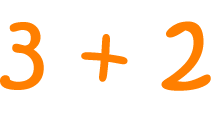# Definition of PlusPlus is another word for "add".

The symbol for plus is $+$.

For example, $8$ elephants plus $3$ elephants equals $11$ elephants.

For example, $3$ apples plus $2$ apples equals $5$ apples.

### Description

The aim of this dictionary is to provide definitions to common mathematical terms. Students learn a new math skill every week at school, sometimes just before they start a new skill, if they want to look at what a specific term means, this is where this dictionary will become handy and a go-to guide for a student.

### Audience

Year 1 to Year 12 students

### Learning Objectives

Learn common math terms starting with letter P

Author: Subject Coach
You must be logged in as Student to ask a Question.